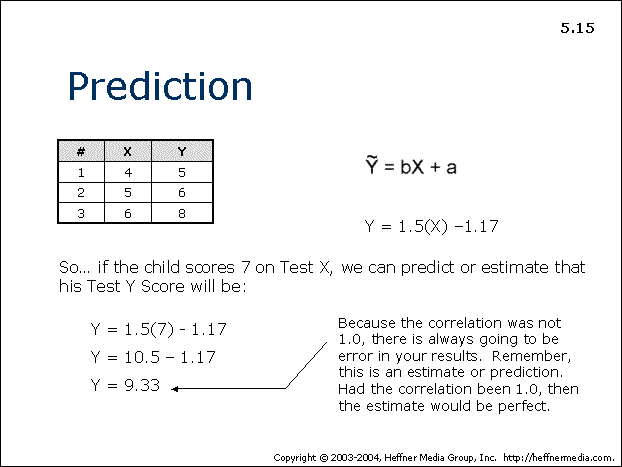# 15: Prediction## Prediction

See Equation Above

Y = 1.5(X) -1.17

So…if the child scores 7 on Test X, we can predict or estimate that his Test Y Score will be:

Y = 1.5(7) – 1.17

Y = 10.5 – 1.17

Y = 9.33*

*Because the correlation was not 1.0, there is always going to be error in your results. Remember, this is an estimate or prediction. Had the correlation been 1.0, then the estimate would be perfect.